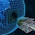### The ring of Numbers

Again: copied from Saurabh Joshi's blog

Problem : Integers from 1 to n are arranged in a circle in some random permutation. Prove that there exist a set of k consecutive integers on the circle sum of which would be at least k(n+1)/2.

Solution :
Highlight the part between the * symbols for the answer.
* Set of k consecutive integers can be uniquely specified by the start position and the direction ( clockwise, or counter clockwise ). We will call it a k-box. Because they are arranged in a circular fashion there are n positions. We will assume clockwise direction. For each of these n position compute sum of its corresponding k-boxes. Sum of all these k-boxes would be kn(n+1)/2. The reason is that \sum_{i=1} ^{n} i = n(n+1)/2. and each number will appear in k different k-boxes.

So on average, each k-box makes a contribution of k(n+1)/2. So there exist at least one k-box which makes contribution of at least k(n+1)/2.

Or, in other words. If none of the k-box can make contribution of at least k(n+1)/2 then the total of all k-boxes would be strictly less than kn(n+1)/2 which is a contradiction. *

1.Consider the k-box with biggest sum, let it be alpha. Break the circle into n/k pieces with one of them being alpha k-box. Sum of these is less than (n/k)*alpha => n(n+1)/2 alpha>k(n+1)/2.

2.Directly, it can be thought like this:
Sum of all integers on the ring is n(n+1)/2. Treating the numbers as variables, the expected value every integer can take is sum/n ,i.e., (n+1)/2. For k consecutive integers, expected sum would be k(n+1)/2. Now, we know this is the mean value and every integer takes a different value (some takes greater than mean, some takes lesser). So, There would definitely exist a combination with sum of at least k(n+1)/2.